Courses

# Introduction to Acceleration Class 11 Notes | EduRev

## Class 11 : Introduction to Acceleration Class 11 Notes | EduRev

The document Introduction to Acceleration Class 11 Notes | EduRev is a part of the Class 11 Course Physics Class 11.
All you need of Class 11 at this link: Class 11

Acceleration
It is the rate of change of velocity with time. The only two ways to accelerate is by changing the speed or change in direction or change both. It is a vector quantity. If the velocity of the object increases with time, its acceleration increases. If the velocity of an object decreases with time, its acceleration is negative.
The motion is uniformly accelerated motion or it non-uniformly accelerated, depending on how the velocity changes with time. It is uniform for a body if the velocity changes by equal amounts in equal intervals and if its velocity changes by unequal amounts, it is non-uniform.
Acceleration=  Change in velocity/time taken

Its unit is m/s²

Constant speed does not guarantee that acceleration is zero. For example, a body moving with constant speed in a circle changes its velocity every instant and hence its acceleration is not equal to zero.

Velocity is a quantity having both magnitude and direction, a change in velocity may involve either or both of these factors. Acceleration may result from a change in speed, a change in direction or changes in both. Like velocity, acceleration can also be positive, negative or zero.

Acceleration Formula

Acceleration is a measure of how quickly the velocity of an object changes. So, the acceleration is the change in the velocity, divided by the time. Acceleration has a magnitude (a value) and a direction. The direction of the acceleration does not have to be the same as the direction of the velocity. The units for acceleration are meters per second squared (m/s2).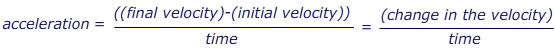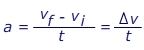a = acceleration (m/s2)
vf = the final velocity (m/s)
vi = the initial velocity (m/s)
t = the time in which the change occurs (s)
Δv = short form for "the change in" velocity (m/s)

Motion in Different Acceleration for Different Time Intervals
Let’s understand this through an example. Suppose, a particle started its motion from rest with an acceleration of 1m/s² for 2s and then continued it for next 1s changing to 2m/s². The distance travelled during this will be:
After 2s the velocity is, v = u+at = 2 m/s

Now, if this is the initial velocity for the second half of the motion, s2=ut+(1/2)at² =3 m
Distance traveled in first half is: s1 = 0+(1/2)at² = 2 m
Hence total distance traveled = s1+s= 5 m

Average Acceleration
It is the change in velocity divided by an elapsed time. For instance, if the velocity of a marble increases from 0 to 60 cm/s in 3 seconds, its average acceleration would be 20 cm/s². This means that the marble’s velocity will increase by 20 cm/s every second.

It is the rate of change of velocity with respect to displacement

Acceleration is a = dv/dt
∴ a = dv/(dx/v)
a = v(dv/dx)

Freefall object experiences an acceleration of g= 9.8m/s² in a downward direction that is towards the center of the earth. In upward direction it is -g = -9.8m/s²

Acceleration of the Velocity-Time graph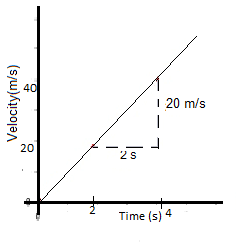In the given graph, a =  (40-20)/(4-2) = 10 m/s2. For a particle it is equal to the slope of a velocity-time graph.

Solved Example:
Q. A stone of mass m is thrown straight upward from the top of a multi-story building with an initial velocity of +15 m/s. Find out the acceleration of stone just after it is thrown?

A. Zero
B. 10m/s² downward
C. 15m/s² upward
D. 15m/s² downward
Answer: B. A body in the air always experiences a gravitational force in a downward direction. Thus the body is in the downward direction with a constant magnitude.

Q. A sports car is travelling at a constant velocity v = 5.00 m/s. The driver steps on the gas, and the car accelerates forward. After 10.0 seconds, the driver stops accelerating and maintains a constant velocity v = 25.0 m/s. What was the car’s acceleration?
The initial velocity is v= 5.00 m/s, in the forward direction. The final velocity is vf = 25.0 m/s in the forward direction. The time in which this change occurred is 10.0 s. The acceleration is in the forward direction, with a value: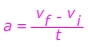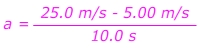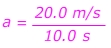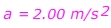The car's acceleration is 2.00 m/s2, forward.

Q. A child drops a rock off of a cliff. The rock falls for 15.0 s before hitting the ground. The acceleration due to gravity is g = 9.80 m/s2. What was the velocity of the rock the instant before it hit the ground?
The rock was released from rest, so the initial velocity is vi = 0.00 m/s. The time in which the change occurred is 15.0 s. The acceleration is 9.80 m/s2. The final velocity must be found, so rearrange the equation: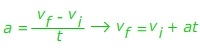v= vi + at
vf = 0.00 m/s +(9.80 m/s2)(15.0 s)
vf = 147 m/s
The rock is falling, so the direction of the velocity is down.

Offer running on EduRev: Apply code STAYHOME200 to get INR 200 off on our premium plan EduRev Infinity!

,

,

,

,

,

,

,

,

,

,

,

,

,

,

,

,

,

,

,

,

,

;